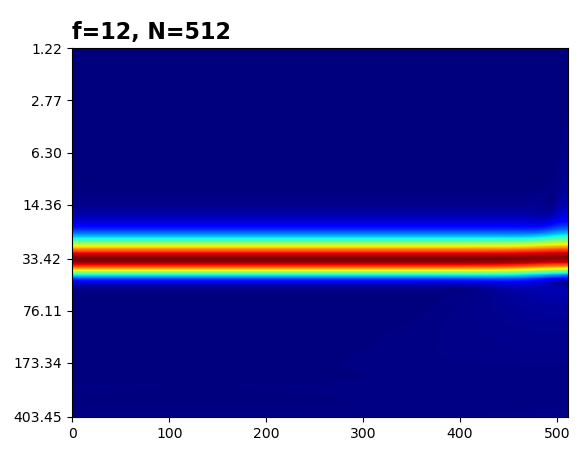# Not showing the right frequency in ssqueezepy CWT plot

I am following the ssqueezepy simple example.

The plot should show f=12 but it shows around 33.1. Is this a bug or have I scaled the y-axis wrong?
2. Also the plot is not a straight plot as it waves at the right end, close to 500.
import numpy as np
from ssqueezepy.visuals import imshow
from ssqueezepy import cwt

def _t(min, max, N):
return np.linspace(min, max, N, False)

def cos_f(freqs, N=128, phi=0):
return np.concatenate([np.cos(2 * np.pi * f * (_t(i, i + 1, N) + phi))
for i, f in enumerate(freqs)])

wavelet = ('morlet', {'mu': 5})
f, N = 12, 512

x = cos_f([f], N=N)
Wx, scales, *_ = cwt(x, wavelet,fs=N)

imshow(Wx, abs=1, yticks=scales, title="f=%d, N=%d" % (f, N), show=1,
cmap='jet')


Note yticks=scales. You need frequencies. Regarding the boundary effect, back then I didn't bother to make a perfect sine CWT: long story short, the padded signal must be a perfect sine, for 'reflect' achieved via endpoint=True. Implemented:If the link doesn't work, here are the only changes:

from ssqueezepy.experimental import scale_to_freq
return np.linspace(min, max, N, True)
freqs = scale_to_freq(scales, wavelet, N, fs=N)
imshow(Wx, abs=1, yticks=freqs, title="f=%d, N=%d" % (f, N), show=1,

• Can't access the link of your implementation. It seems that one should convert the scales to frequency and I use the scale2frequency code of ssquezepy in the latest version. It works for me. Jun 21, 2022 at 7:21